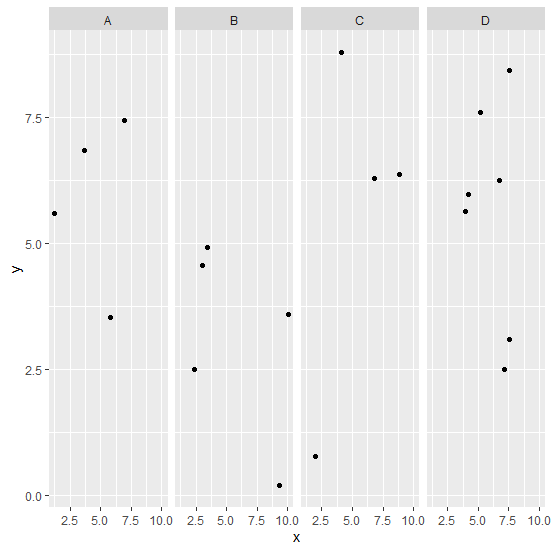# How to create scatterplot for factor levels in an R data frame?

To create a scatterplot for factor levels, we can use facet_grid function of ggplot2 package. For example, suppose we have a factor column in a data frame df defined as F and numerical columns defined as x and y then the scatterplot for the factor levels can be created as −

ggplot(df,aes(x,y))+geom_point()+facet_grid(~Factor)

## Example

Live Demo

Consider the below data frame −

set.seed(1251)
Factor<−as.factor(sample(LETTERS[1:4],20,replace=TRUE))
x<−rnorm(20,5,2)
y<−rnorm(20,5,3)
df<−data.frame(Factor,x,y)
df

## Output

   Factor    x    y
1 A 1.252323 5.5936677
2 A 6.920217 7.4327577
3 D 3.947190 5.6392972
4 B 3.448531 4.9231640
5 C 2.015025 0.7748935
6 B 10.064797 3.6023493
7 A 3.693243 6.8432984
8 D 6.689182 6.2388841
9 C 6.824572 6.2926420
10 B 9.344411 0.2018111
11 D 7.534430 3.1049284
12 B 3.001813 4.5642253
13 D 7.539027 8.4313003
14 B 2.357861 2.5036640
15 D 5.213361 7.5924318
16 C 8.808244 6.3627349
17 D 7.160700 2.4980811
18 C 4.099854 8.7798943
19 D 4.210711 5.9739498
20 A 5.805695 3.5373963

library(ggplot2)
ggplot(df,aes(x,y))+geom_point()+facet_grid(~Factor)